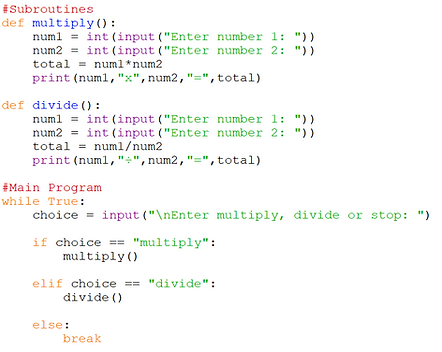## Subroutines

A subroutine is a section of code that can be re-used several times in the same program.

It is separate from the main code and has to be ‘called’ upon.

Subroutines are designed to be repeated, and they have three key benefits:

1. Subroutines make programs easier to read.

2. They reduce the duplication of code.

3. Complex problems are broken down into smaller chunks.

There are two types of subroutines: procedures and functions.

• A procedure just executes commands, such as printing something a certain number of times.

• A function produces information by receiving data from the main program and returning a value to the main program.

For example, a function could take the radius of a sphere from the main program, calculate a sphere’s area and return the value of the area to the main program.

A function generally requires parameters to work – these are the values to be transferred from the main program to the subroutine.

## Procedures

A procedure is a type of subroutine that runs independently of the main program.

A subroutine must be defined at the top of the program before the main code by typing def and the name of the subroutine.

In the example below I have created a procedure to calculate the multiplication of two numbers and a separate procedure for the division.The main program starts beneath the subroutines, against the left side of the editor.

I have created a while true loop that asks the user if they want to multiply, divide or stop the program.

If they choose to multiply, the multiply subroutine is called. This initiates that subroutine then returns to the main program when it is complete.

If they choose to divide, the divide subroutine is called instead. Typing stop will break (end) the loop.Here you can see the two parts of the program put together.

Subroutines must be written first, with the rest of the program underneath.Subroutines can be called in any order. Below I have run the program and divided then multiplied before breaking the loop:

1. Create a procedure called hello that just prints “Hello there!”.

In the main program create a for loop that calls the procedure 10 times.

You must use a procedure.

2. Create a program with two procedures. The addition procedure allows the user to add two numbers together. The subtraction procedure allows the user to take a number away from another. Use a while true loop in the main program and a break to stop the loop.

Example solution for #1:## Local & Global Variables

Programming languages use local variables and global variables.

A global variable can be used anywhere in the program.

A local variable can only be used in the subroutine it is created in.

I have adapted the multiply / divide program below to use global variables by stating the global command and the name of the variables in each subroutine. This allows me to ask the user to enter numbers in the main program.